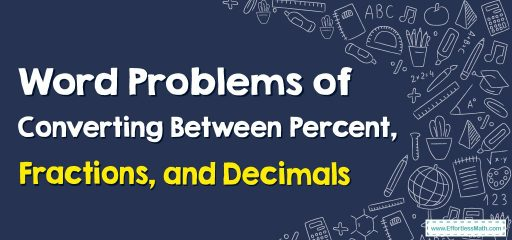# Word Problems of Converting Percent, Fractions, and Decimals

In this step-by-step guide, you will learn how to solve Word Problems related to Converting Between Percent, Fractions, and Decimals.## A step-by-step guide

To convert a percent to a fraction, divide the percent by 100 and add the percent sign (%). For example, 25% can be written as 25/100 or 1/4.

To convert a fraction to a percent, divide the numerator by the denominator and multiply by 100. For example, 1/4 can be written as 25%.

To convert a decimal to a percent, multiply the decimal by 100 and add the percent sign (%). For example, 0.25 can be written as 25%.

To convert a percent to a decimal, divide the percent by 100. For example, 25% can be written as 0.25.

To convert a decimal to a fraction, you can use the following steps:

1. Write the decimal as a fraction with a denominator of 1 followed by as many zeroes as the decimal has digits. For example, 0.25 would be written as 25/100.
2. Simplify the fraction by dividing both the numerator and denominator by their greatest common factor (GCF).

To convert a fraction to a decimal, divide the numerator by the denominator. For example, 1/4 can be written as 0.25

### Word Problems of Converting Between Percent, Fractions, and Decimals – Examples 1

Bettie is helping her mother clean out the basement. She finds a bolt that is 3/5 of a foot in diameter.
Write $$\frac{3}{5}$$ of a foot as a decimal and percent.
Solution:
Divide 3 by 5 to write as a decimal. $$3÷5=0.6$$
Multiply $$\frac{3}{5}$$ by 100 to write it as a percent. $$\frac{3}{5}×100=\frac{3}{5}×\frac{100}{1}=\frac{300}{5}=60$$
So, $$\frac{3}{5}$$ is equal to 0.6 and $$60%$$

### Word Problems of Converting Between Percent, Fractions, and Decimals – Examples 2

Carol is painting his bedroom. He uses 45% of a gallon.
Write 45% of a gallon as a fraction.
Solution:
45% is 45 parts out of 100. So, as a fraction, it is $$\frac{45}{100}$$. Simplify $$\frac{45}{100}=\frac{45÷5}{100÷5}=\frac{9}{20}$$.
To write 45% as a decimal, divide 45 by 100. $$45÷100=0.45$$.

### What people say about "Word Problems of Converting Percent, Fractions, and Decimals - Effortless Math: We Help Students Learn to LOVE Mathematics"?

No one replied yet.

X
30% OFF

Limited time only!

Save Over 30%

SAVE $5 It was$16.99 now it is \$11.99